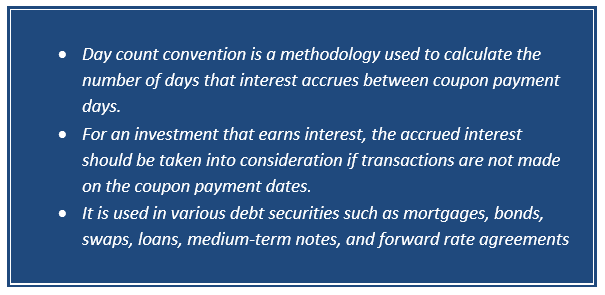# Day Count Convention

What is Day Count Convention?

Day Count Convention also refers as day count fraction or day count. It is a methodology used to calculate the number of days that interest accrues between coupon payment days. In other words it calculates the number of days between two dates. It is used in various debt securities such as mortgages, bonds, swaps, loans, medium-term notes and forward rate agreements (FRAs).

Day Count Convention was invested to standardize the interest calculating methodology, to avoid disputes and provide transparency and uniformity as months and years have different number of days and calculating interest for different period can be complex, complicated, and less reliable.

For an investment that earns interest, the accrued interest should be taken into consideration if transactions are not made on the coupon payment dates.  The day count may be used to determine an accrual factor stating the days of an accrual period and how to count the days of an entire coupon period.The interest on money market instrument and floating rate is calculated on an actual/360-day count conversion while bonds and notes issued by the US Treasury uses the Actual/Actual basis and corporate bonds use the 30/360 day count method.

The International Swap Dealer Association providers various rules and definitions for using the day count convention. The London Inter Bank Offered Rate (LINOR) is the most commonly used interest rate, which is posted daily at 11:45 A.M. (London Time).

How to calculate Day Count Convention?

To determine interest for a particular payment period, the following formula is used:

Interest Amount = Principal/Notional amount x interest rate x Day count fraction

Where:

• The interest amount and principal/notional amount are expresses as an amount of currency such as US dollar or Euros.
• Interest rate is expressed in percentage.
• The day count fraction is a fraction in which both numerator and denominator are calculated by the day count convention specified by the parties in financial contract.

The day count convention is calculated as:

Day Count Convention = Number of days in the accrual period/ Number of days in the year

The day count convention consists of two components. The first component calculated the number of days in a month to calculate the days in an accrual period and the second component calculate the days in a year or a full coupon period.

For example: a Bond uses 30/360 day count convention means the number of days is counted on the basis of 30 days per month and 360 days per year.

Types of Day Count Conventions

The Day Count Convention depends upon the types of securities, country of issuance, type of interest rate (fixed or floating), issuers and other factors.

The Two main types of day count convention include:

• Actual Method

The Actual method considers the actual number of days during the accrual period or the coupon period.

The Actual/360 method is used by the money market instrument. In this the actual number of days from the effective date to the terminating date is used for the accrual period. It calculates the daily interest for 360 day per year and then multiplies it by the actual number of days in each time period.

The Actual/Actual method uses the actual number of days in each month and year to calculate the daily interest and then multiply it by the actual number of days in each period. The method is commonly used for US treasury bonds.

The Actual/360 method determines the daily interest by taking 360 days in a year and then multiplies it by the actual number of days in each time period.  This method is mostly used for money market instruments.

Further the Actual/365L and Actual/365 method are some of the less common actual methods. The Actual/365L consider leap years, uses 366 days in a year for leap years and 365 for non-leap years and the Actual/365 method divides the actual number of accrual days by 365.

• 30/360 Method

In 30/360 method, determining actual days is not needed as it determines the denominator as 360 that is composed of twelve 30 day months. In case the start or end day of an accrual period has 31 days, the date will be moved to 30 days in a month and then multiplies it by 30 days. This method is commonly used for corporate bonds.

The 30/365 method uses 365 days in a year to determine daily interest and then it is multiplied by 30. The other method includes 30E+/360 and 30E/360 and so on.

If the first day of the accrual period in 30E+/360 method has 31 days, it will be moved as 30 days, however if the last day has 31 days, it will be moved as the first day of the next month.

If the end day of the accrual period in 30E/360 method falls in February the actual number of days will be counted instead of extending it to 30 days.

##### Top ASX Listed Companies

Definition:
We use cookies to ensure that we give you the best experience on our website. If you continue to use this site we will assume that you are happy with it. OK